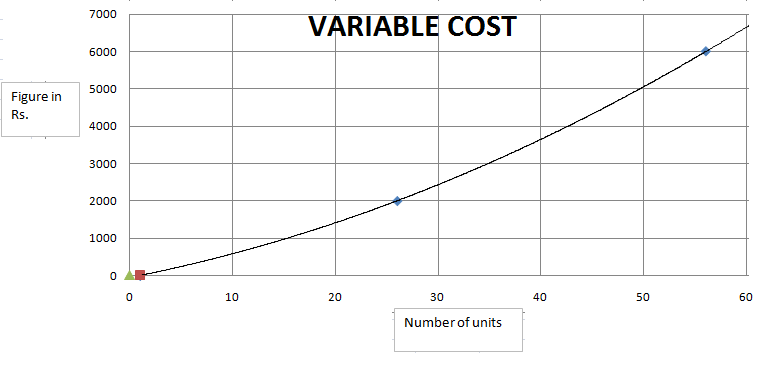### CAT 2000 Question Paper Question 33

Instructions

Answer these questions based on the following Information:

ABC Ltd. produces widgets for which the demand is unlimited and they can sell all of their production. The graph below describes the monthly variable costs incurred by the company as a function of the quantity produced. In addition, operating the plant for one shift results in a fixed monthly cost of Rs. 800. Fixed monthly costs for second shift operation are estimated at Rs. 1200. Each shift operation provides capacity for producing 30 widgets per month.
Note: Average unit cost, AC = Total monthly costs/monthly production, and Marginal cost MC is the rate of change in total cost for unit change in quantity produced.Question 33

# ABC Ltd. is considering increasing the production level. What is the approximate marginal cost of increasing production from its July level of 40 units.

Solution

We know that  MC is the rate of change in total cost for unit change in quantity produced. SO for 40 units total cost = 2000+3700 = 5700 and for 50 units  = 2000 + 5000 = 7000. So for additional 10 units change in total costs is 1300 . So for 1 unit we have MC = 130.